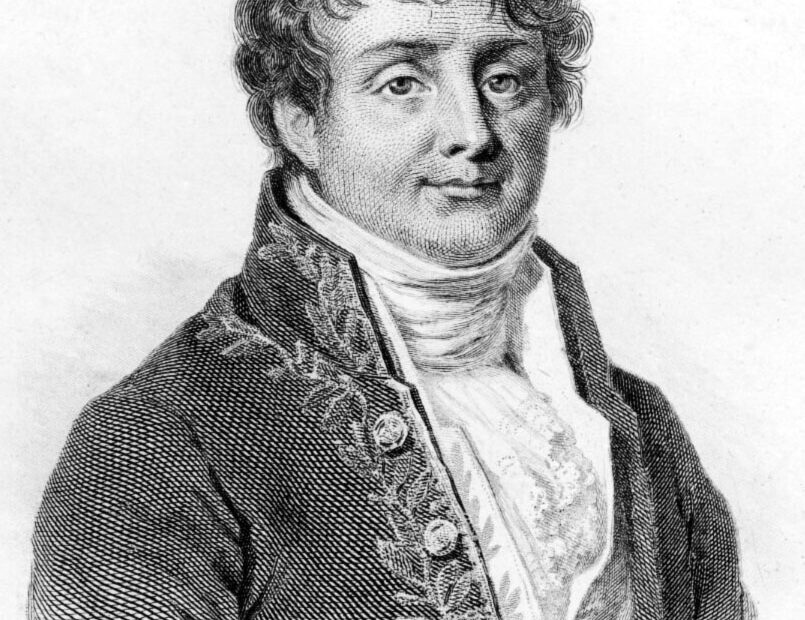# Lectures on Signals and Systems

• ProjectThis are the lectures that I gave in first semester of 2020 for Los Andes University, Chile. That year the lectures where completely online for the first time due to the COVID-19 pandemic. I start given this class on the first semester of 2017, and since then I have myself learn a lot by teaching. In general this is highly mathematical course, a base background for the Electrical Engineering Specialty and even other disciplines. The program and methodology was updated through the years, center on examples and practical applications, making it more approachable for students.

Even though lectures are in Spanish, I put a short fragment of the program here in English. Also, some of the supplemental material given to the students.

## Program

The course covers some of the fundamental mathematical tools used throughout the electrical specialty for signal analysis and system design. Emphasizing applications, the course is presented through practical examples. At the heart of the course are the Fourier transform for frequency analysis and the Laplace transform for representing linear systems. Then, the techniques learned are extended to the digital world for the analysis of discrete signals and discrete systems. The course is carried out, for simplicity and historical reasons, mainly in one dimension (with time as the independent variable). Despite the above, given the large number of applications, at the end of the course there is the option of generalizing to two dimensions for image processing.

### Objectives and applications

1. In audio signals it is natural to study frequency analysis of vibrations for useful information. Understand the functioning of the ear, microphone, and the special nature of musical notes.
2. Design of electrical and mechanical filters using passive elements (resistors, capacitors, inductors, springs and dampers). Study their behavior in frequency (characterize a low pass, high pass or band pass with their respective cutoff frequencies) and the resonance phenomenon.
3. Concept of modulation and design of an AM radio. Introduction to communications.
4. Characterize linear systems, study their stability and subsequent automatic control systems using feedback (for example, for the design of a magnetic levitator by attraction).
5. Sampling theory operation to go from a continuous to a discrete signal, determine the optimal sampling rate, perform digital processing and reconstruction.
6. Understand the principles of digital signal processing. Basic discrete filter programming, cross-correlation for pattern detection, and a guitar tuner for projects.
7. Advanced applications in two dimensions. (optional)